# 高维数据降维中的PCA与CUR分解对比分析PCA and CUR Decomposition Analysis in Dimensionality Reduction of High-Dimensional Data

DOI: 10.12677/SA.2020.92028, PDF, HTML, XML, 下载: 109  浏览: 181  科研立项经费支持

Abstract: In the era of big data, there are many ways to reduce the dimension of high-dimensional data. Among them, the linear dimension reduction method is the most typical method in PCA and CUR decomposition method, but at present, using the two methods on the research achievements of high-dimensional data dimension reduction is not enough. Therefore, through the discussion and research of the principal component analysis and CUR decomposition method, this paper analyzes the use conditions and practical effects of the two methods. It is concluded that: with the traditional principal component analysis method of the matrix decomposition, in terms of feature selection, CUR decomposition method not only has high accuracy, but also has good interpretability. In terms of matrix recovery, CUR matrix decomposition method has high stability and accuracy, and its accuracy can sometimes reach more than 90%. Therefore, I think CUR matrix decomposition has good application value, and it is worth using CUR matrix decomposition method to reduce the dimension of high-dimensional data.

1. 引言

2. 理论模型与方法论

2.1. 理论模型

${\left\{\left(\lambda \right){W}_{I}{}^{J}\right\}}_{i=1,2,3,\cdots ,{2}^{n}-1;j=1,2,3,4,\cdots ,m}$ (1)

${\left\{\left(\lambda \right){W}_{I}{}^{J}\right\}}_{i=1,2,3,\cdots ,{2}^{n}-1;j=1,2,3,4,\cdots ,m}$ (2)

2.2. 方法论

2.2.1. PCA方法论

PCA方法是一类经典的降维方法，其目的在于用一组较少的不相关变量来代替原始数据集中的变量(往往维数较高)，同时保证原始数据集中的信息尽可能多的被解释，称这些提取出来的较少的不相关变量为主成分。其方法论为：最大方差理论。具体表现为寻找一组基，使得所有数据变换为在这组基上的坐标表示后，其方差值最大。形象的可以理解为数据在这组基上的投影尽可能的分能的分散，因此，方法论可以归纳为求解最优化问题：

$Y=xl$ (4)

2.2.2. CUR分析方法

CUR分解方法主要就是通过将一个矩阵变为三个矩阵CUR矩阵，CUR特征选择方法是计算统计影响力，先计算一下每一列和每一行特征的得分值，然后根据我们已知的得分的大小来决定是否选择这行(列)，通过这一方法，我们可以将不需要的数据去掉，保留有用的数据，得到可以代表其特征的集合，通过这样的集合就可以快速且容易地的分析原数据所具备的特征，本文通过CUR矩阵得到恢复矩阵 $\stackrel{⌢}{A}$，恢复矩阵： $A\approx CUR=\stackrel{⌢}{A}$

$\text{ERROR}=\frac{{‖A-CUR‖}_{F}}{{‖A‖}_{F}}\ast 100%$ (5)

3. 基本算法

3.1. PCA基本算法

3.2. CUR基本算法

${p}_{i}=\frac{{\sum }_{j=1}^{n}\text{ }\text{ }{A}_{ij}}{{\sum }_{i=1}^{m}\text{ }\text{ }{\sum }_{j=1}^{n}\text{ }\text{ }{A}_{ij}},\text{\hspace{0.17em}}i=1,2,\cdots ,m$ (6)

${q}_{j}=\frac{{\sum }_{i=1}^{m}\text{ }\text{ }{A}_{ij}}{{\sum }_{i=1}^{m}\text{ }\text{ }{\sum }_{j=1}^{n}\text{ }\text{ }{A}_{ij}},j=1,2,\cdots ,n$ (7)Table 2. Row and row selection algorithmTable 3. Construction of algorithm 2 matrix UTable 4. Algorithm 3 USES CUR for feature selection and recovery

4. 实证分析

4.1. 研究对象选取

4.2. PCA算法实现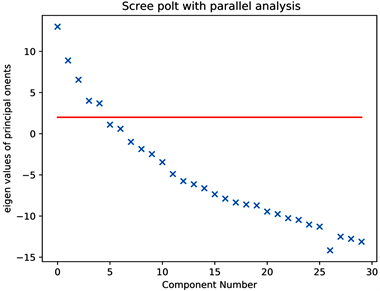Figure 1. Principal component diagram

$\underset{f\in F}{\mathrm{min}}\frac{1}{n}W\left({y}_{i},f\left({x}_{i}\right)\right)+\lambda ‖f‖$ (8)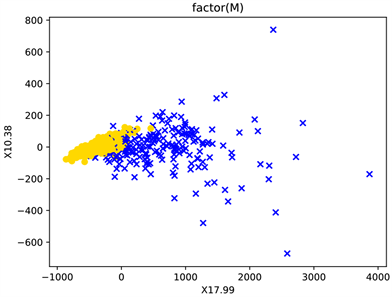Figure 2. Classification of data by maximum two variables of covariance matrix eigenvalue of experimental data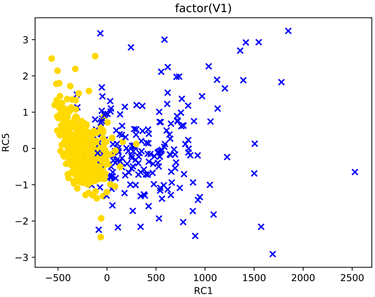Figure 3. PCA classification of experimental data

4.3. CUR矩阵恢复

$\text{ERROR}\left(\text{%}\right)=\frac{{‖A-C\cdot U\cdot R‖}_{F}}{{‖A‖}_{F}}$ (9)

4.3.1. 实验构造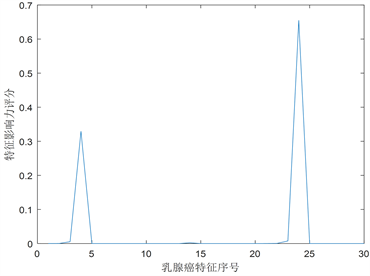Figure 4. Influence distribution of 32 characteristics of breast cancerFigure 5. Influence distribution of 569 patients with breast cancer

4.3.2. c和r值的确定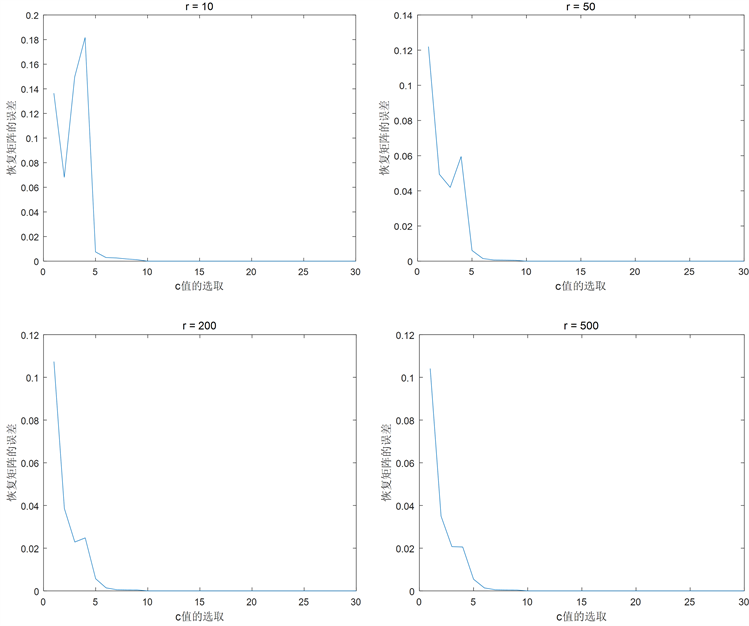Figure 6. Influence of the selection of constant value on the error of response matrix

5. 结论

CUR算法在选取行列的时候是根据行列的实际情况比较智能地进行选择，在研究大数据时，我们可以理解为在选择行和列的时候，CUR算法判断矩阵列的统计影响力来判断特征的显著程度，然后根据显著的特征从电影列中进行抓取，所以构造出的C(R)矩阵也反映了癌症良性恶性的现实特征，并且因为C(R)矩阵是由真实的行列构成，因此将C(R)矩阵中的行列数据和Wdbc数据集相比对就能够确定细胞核特征和良性恶性的具体特征，但是在软件操作上CUR比PCA复杂得多，所以如果不是研究高维稀疏的数据集建议用PCA降维方法，但在解释性方面，由于CUR分解方法是在原始数据集中提取出来的，所以其可解释性很强 。

  Bobadilla, J., Ortega, F. and Hernando, A. (2013) Recommender Systems Survey. Knowledge-Based Systems, 46, 109-132. https://doi.org/10.1016/j.knosys.2013.03.012  林海明. 对主成分分析法运用中十个问题的解析[J]. 统计与决策, 2007(16): 16-18.  雷恒鑫, 刘惊雷. 基于行列联合选择矩阵分解的偏好特征提取[J]. 模式识别与人工智能, 2017, 30(3): 279-288.  雷恒鑫, 刘惊雷. 利用CUR矩阵分解提高特征选择与矩阵恢复能力[J]. 计算机应用, 2017, 37(3): 640-646.  Kumar, R., Verma, B.K. and Rastogi, S.S. (2014) Social Popularity Based SVD++ Recommender System. International Journal of Computer Applications, 87, 33-37. https://doi.org/10.5120/15279-4033  张梦阳. 矩阵分解的常用方法[J]. 成才之路, 2012(36): 39-39.  Jia, Y., Zhang, C., Lu, Q., et al. (2014) Users’ Brands Preference Based on SVD++ in Recommender Systems. Proceedings of the 2014 IEEE Workshop on Advanced Research and Technology in Industry Applications, Ottawa, ON, 29-30 September 2014, 1175-1178.  王群英. 矩阵分解方法的探究[J]. 长春工业大学学报, 2011, 32(1): 95-101.  Xu, F., Gu, G., Kong, X., et al. (2016) Object Tracking Based on Two-Dimensional PCA. Optical Review, 23, 231-243. https://doi.org/10.1007/s10043-015-0178-2  Drineas, P., Mahoney, M.W. and Muthukrishnan, S. (2008) Relative-Error CUR Matrix Decompositions. SIAM Journal on Matrix Analysis and Applications, 30, 844-881. https://doi.org/10.1137/07070471X  李明. 基于矩阵分解理论学习的数据降维算法研究[D]: [硕士学位论文]. 大连: 辽宁师范大学, 2011.